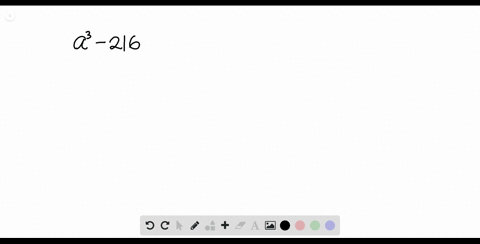Enroll in one of our FREE online STEM summer camps. Space is limited so join now!View Summer Courses### Factor each polynomial. If a polynomial cannot be…

01:18Other Schools

Need more help? Fill out this quick form to get professional live tutoring.

Get live tutoring
Problem 27

Factor each polynomial. If a polynomial cannot be factored, write prime. Factor out the greatest common factor as necessary.
$$9 p^{2}-24 p+16$$

$9 p^{2}-24 p+16=(3 p-4)^{2}$

## Discussion

You must be signed in to discuss.

## Video Transcript

we're asked to factor this expression here. Not that the leading coefficient is not one. So that means we need to multiply our leading coefficient with our constant term. So that would be nine times 16 and that is equal to 144. So now we need to find two factors of 144. I multiply together to be 1 44 and add together to be negative 24. So the negative sighing tells us that bull's both factors have to be negative. So that would be negative. 12 and negative 12. So now what we can do is we've ovary, right, this expression. But we will have the middle term being split up into two terms. So that will be 12. Yeah, 12 p. And then we will look at this two terms out of time. So from our 1st 2 terms, we can factor it out a three peat, and that means we're left with three p minus four form our 2nd 2 terms. We can factor out a negative four, and that means where left was three p minus four. So, as you can see, both of these two terms have the factor three p minus four. So that means we can factor that apt. So that will be three p minus four and three p minus for which is from here and here. So now, since both factors are the same, we can re write. This has three p minus four all squared.